## Types of Graphs-

Before you go through this article, make sure that you have gone through the previous article on various Types of Graphs in Graph Theory.

We have discussed-

• A graph is a collection of vertices connected to each other through a set of edges.
• The study of graphs is known as Graph Theory.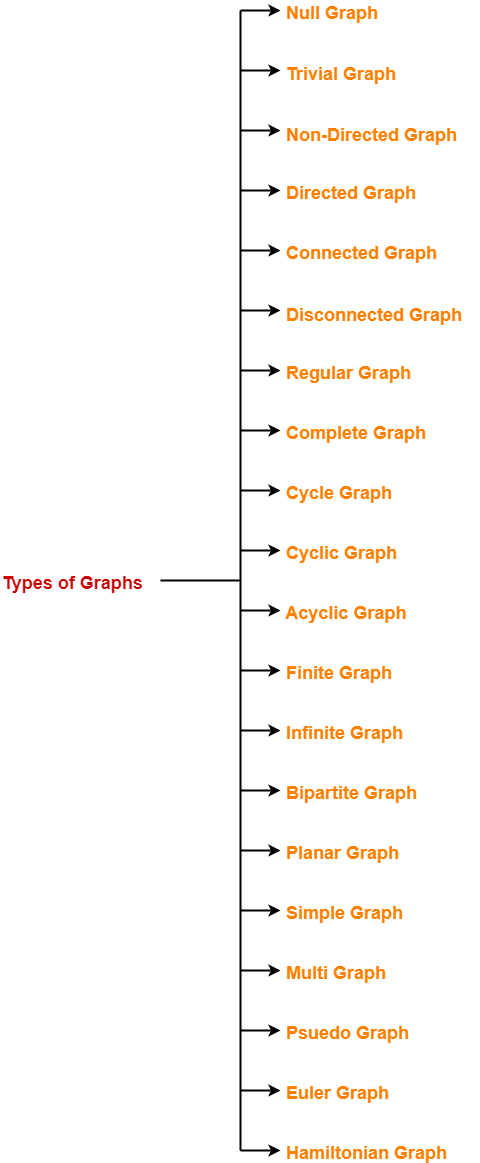## Hamiltonian Graph-

A Hamiltonian graph may be defined as-

 If there exists a closed walk in the connected graph that visits every vertex of the graph exactly once(except starting vertex) without repeating the edges,then such a graph is called as a Hamiltonian graph.ORAny connected graph that contains a Hamiltonian circuit is called as a Hamiltonian Graph.

### Hamiltonian Graph Example-

The following graph is an example of a Hamiltonian graph-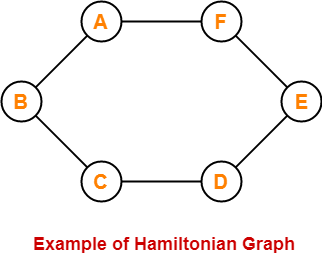Here,

• This graph contains a closed walk ABCDEFA.
• It visits every vertex of the graph exactly once except starting vertex.
• The edges are not repeated during the walk.
• Therefore, it is a Hamiltonian graph.

Alternatively, there exists a Hamiltonian circuit ABCDEFA in the above graph, therefore it is a Hamiltonian graph.

## Hamiltonian Path-

• If there exists a walk in the connected graph that visits every vertex of the graph exactly once without repeating the edges, then such a walk is called as a Hamiltonian path.

OR

• If there exists a Path in the connected graph that contains all the vertices of the graph, then such a path is called as a Hamiltonian path.

### NOTE

In Hamiltonian path, all the edges may or may not be covered but edges must not repeat.

### Hamiltonian Path Examples-

Examples of Hamiltonian path are as follows-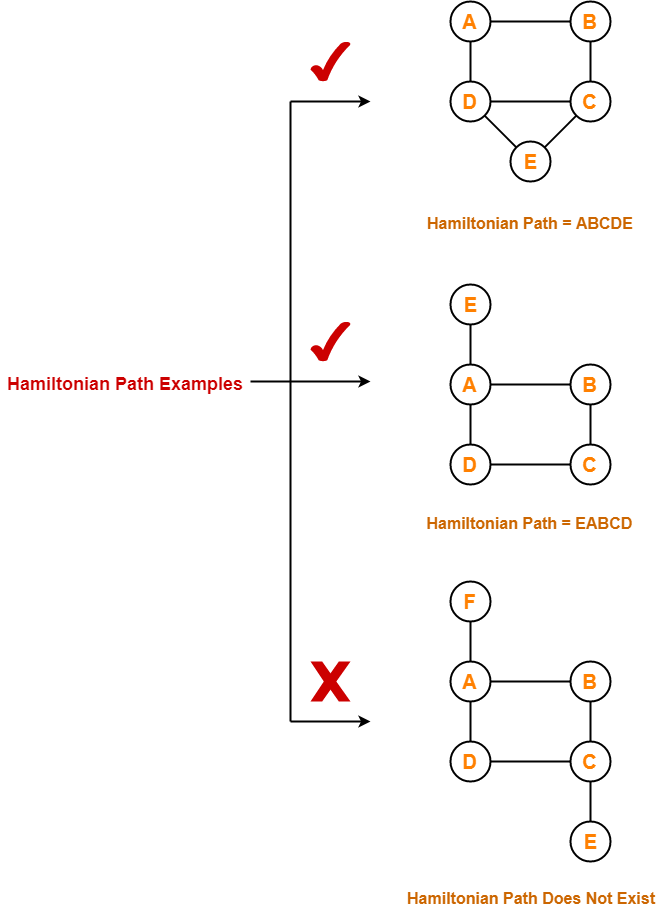## Hamiltonian Circuit-

Hamiltonian circuit is also known as Hamiltonian Cycle.

• If there exists a walk in the connected graph that visits every vertex of the graph exactly once (except starting vertex) without repeating the edges and returns to the starting vertex, then such a walk is called as a Hamiltonian circuit.

OR

• If there exists a Cycle in the connected graph that contains all the vertices of the graph, then that cycle is called as a Hamiltonian circuit.

OR

• A Hamiltonian path which starts and ends at the same vertex is called as a Hamiltonian circuit.

OR

• A closed Hamiltonian path is called as a Hamiltonian circuit.

### Hamiltonian Circuit Examples-

Examples of Hamiltonian circuit are as follows-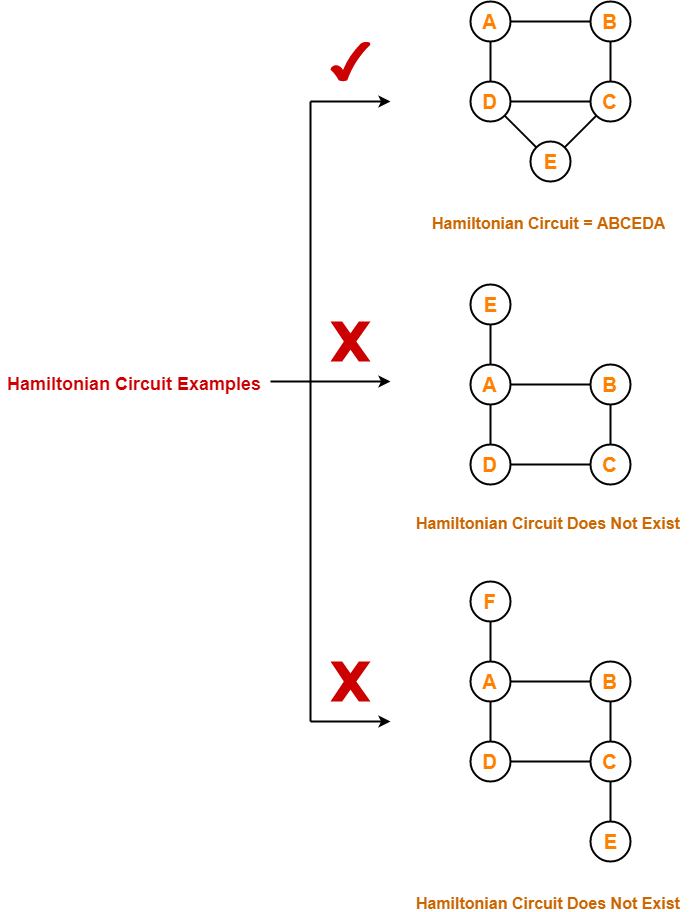## Important Notes-

• Any Hamiltonian circuit can be converted to a Hamiltonian path by removing one of its edges.
• Every graph that contains a Hamiltonian circuit also contains a Hamiltonian path but vice versa is not true.
• There may exist more than one Hamiltonian paths and Hamiltonian circuits in a graph.

## Problems-

Which of the following is / are Hamiltonian graphs?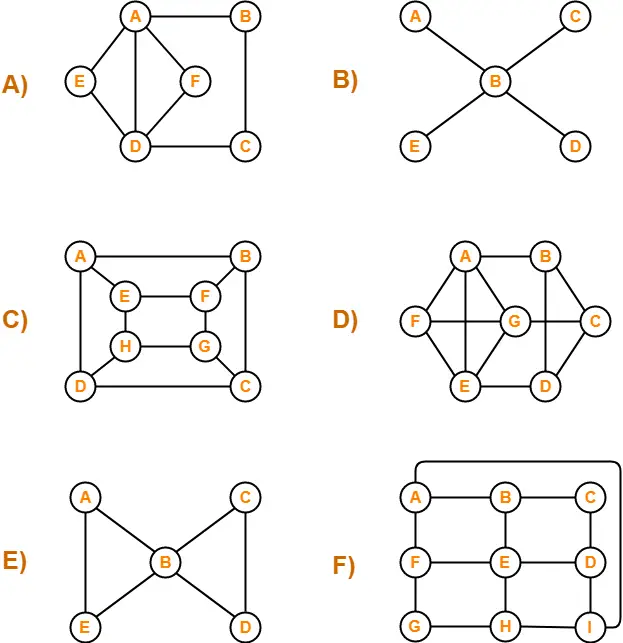## Solutions-

### A)

The graph neither contains a Hamiltonian path nor it contains a Hamiltonian circuit.

Since graph does not contain a Hamiltonian circuit, therefore It is not a Hamiltonian Graph.

### B)

The graph neither contains a Hamiltonian path nor it contains a Hamiltonian circuit.

Since graph does not contain a Hamiltonian circuit, therefore It is not a Hamiltonian Graph.

### C)

The graph contains both a Hamiltonian path (ABCDHGFE) and a Hamiltonian circuit (ABCDHGFEA).

Since graph contains a Hamiltonian circuit, therefore It is a Hamiltonian Graph.

### D)

The graph contains both a Hamiltonian path (ABCDEFG) and a Hamiltonian circuit (ABCDEFGA).

Since graph contains a Hamiltonian circuit, therefore It is a Hamiltonian Graph.

### E)

The graph neither contains a Hamiltonian path nor it contains a Hamiltonian circuit.

Since graph does not contain a Hamiltonian circuit, therefore It is not a Hamiltonian Graph.

### F)

The graph contains both a Hamiltonian path (ABCDEFGHI) and a Hamiltonian circuit (ABCDEFGHIA)

Since graph contains a Hamiltonian circuit, therefore It is a Hamiltonian Graph.

To gain better understanding about Hamiltonian Graphs in Graph Theory,

Watch this Video Lecture

Next Article- Bipartite Graph

Get more notes and other study material of Graph Theory.

Watch video lectures by visiting our YouTube channel LearnVidFun.